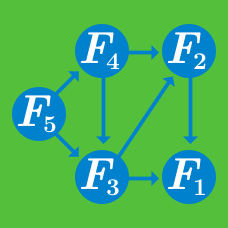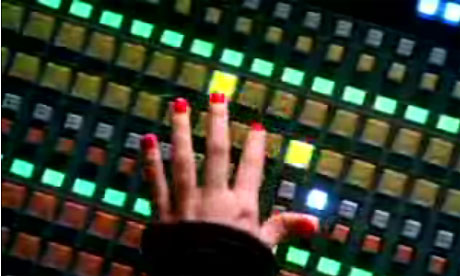Computer Science

# Dynamic Programming: Level 4 ChallengesCandice enjoys candy like any other girl her age. One day, she asks her mother to lend her money to buy candy.

Her mother agrees and lends her $\40$ but specifies a few rules first.

• Candice needs to use all $\40$ and should not have any remaining money.
• Her mother doesn't want her to get carried away so Candice can buy a minimum of 1 and a maximum of 10 candies in total.
• Candice can buy as many of each type of candy she wants provided the above conditions are satisfied.

She agrees and heads over to the candy shop.Over there she notices that the shop offers candies of 4 different types, which are priced at $\1 , \2, \5 \text{ and } \10$ respectively.

If Candice picks each candy one at a time, In how many ways can Candice make her selection?

Details and Assumptions

• As an explicit example, Candice can buy 4 candies of $\10$ each so that she would be left with no money extra.
• Order of purchase matters. If Candice buys the candies as $(5,5,10,10,10)$ and $(5,10,10,10,5)$ both should be considered.
###### Check out Candice's Other Adventures!

Several vaults were broken into last night! Police have strong evidence to believe that the culprit was the infamous Algorithmic Burglar - an efficient and swift robber.

7 different vaults were robbed. Police discovered that the vaults are missing the following amounts of metal: 1739 lbs, 72 lbs, 212 lbs, 55 lbs, 511 lbs, 1239 lbs, and 99 lbs.

Here are the weights and dollar values for the different bars in the vaults:

  1 2 3 4 5 6 7 8 9 10 11 12 Material Weight Value Gold 2 lbs $57 Platinum 5 lbs$191 Silver 14 lbs $417 Expensivium 17 lbs$231 Rhodium 19 lbs $741 Osmium 13 lbs$139 Aluminum 1 lbs $28 Silicon 3 lbs$117 Iron 5 lbs $13 Titanium 11 lbs$9 Potassium 19 lbs \$18 

Before the robbery, each vault contained at least 2000 lbs of each metal. Assume the burglar stole whole bars, not fractions of a bar.

Let V be the greatest possible value in dollars of the stolen material. What are the last three digits of V?Jenny is the operator of a large garbage disposal machine. It is a fairly simple machine and constitutes of only three buttons: One $\color{#20A900} {\text{green}}$ button, one $\color{#3D99F6} {\text{blue}}$ button and one $\color{#D61F06} {\text{red}}$ button.

Given that initially $n$ items are put in the machine, it will only destroy garbage in the three following ways:

• The $\color{#20A900} {\text{green}}$ button, if pressed destroys $\frac{1}{2}$ of the items,leaving $\frac{n}{2}$ of the items, but it only works if the number of items $n$ is divisible by $2.$
• The $\color{#3D99F6} {\text{blue}}$ button,if pressed destroys $\frac{2}{3}$ of the items,leaving $\frac{n}{3}$ items, but it only works if the number of items $n$ is divisible by $3.$
• The $\color{#D61F06} {\text{red}}$ button always works and destroys only $1$ item leaving $n-1$ if pressed.

Since Jenny is a lazy operator she wants to minimize the number of times she presses the buttons? What is the minimum number of times Jenny has to press the buttons in order to completely destroy $466559$ items?

Examples
As explicit examples for $n=10$ items Jenny would have to do $4$ button presses ($\color{#D61F06} {\text{red}}$,$\color{#3D99F6} {\text{blue}}$, $\color{#3D99F6} {\text{blue}}$,$\color{#D61F06} {\text{red}}$) to minimize the number of presses as shown below.
$10-1=9 \longrightarrow$ $9\diagup 3=3 \longrightarrow$$3\diagup3=1 \longrightarrow$$1-1=0$

For $n=6$ items the optimal solution is $3$ presses ($\color{#3D99F6} {\text{blue}}$, $\color{#20A900} {\text{green}}$,$\color{#D61F06} {\text{red}}$) or ($\color{#3D99F6} {\text{blue}}$,$\color{#D61F06} {\text{red}}$,$\color{#D61F06} {\text{red}}$)
$6\diagup 3 \longrightarrow 2 \diagup 2 \longrightarrow 1 - 1=0$ or $6\diagup 3 \longrightarrow 2 - 1 \longrightarrow 1 - 1=0$

×